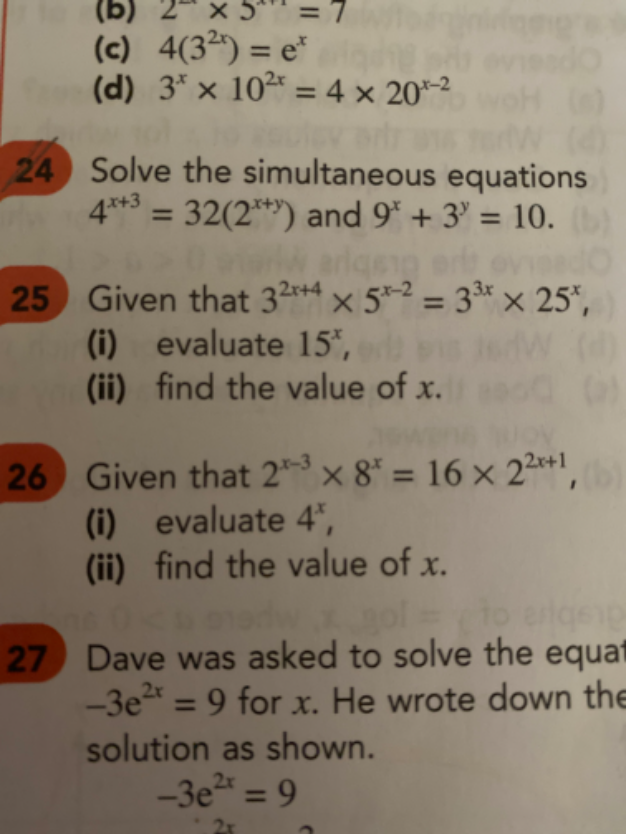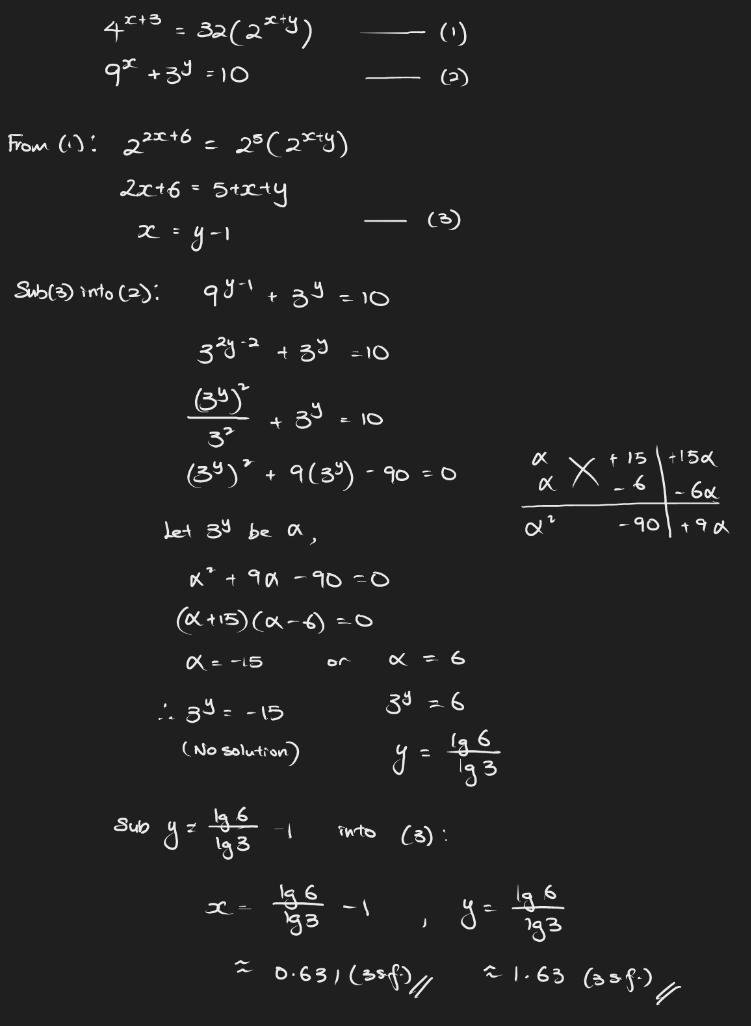# QuestionPlease check if the answer for Question 24 is x = 1 and y = 0?   If not, please provide the answer key.

Question 25:

(a)   32x+4 x 5x-2  =  33x x 52x

32x+4                         52x

——-           =        ———-

33x                                  5x-2

32x+4-3x       =        52x-x+2

34-x               =        5x+2

34 3-x              =        5x 52

5x 3x             =      34 /  5  = 81 / 25

15x                 =      3.24

(b)         x log 15           =     log 3.24

x                 =  log 3.24  / log 15   =  0.434 ( to 3 dec place)